### IMO Shortlist 2002 problem G4

Kvaliteta:
Avg: 0,0
Težina:
Avg: 7,0
Circles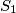$S_1$ and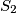$S_2$ intersect at points$P$ and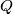$Q$. Distinct points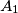$A_1$ and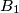$B_1$ (not at$P$ or$Q$) are selected on$S_1$. The lines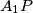$A_1P$ and$B_1P$ meet$S_2$ again at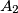$A_2$ and$B_2$ respectively, and the lines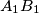$A_1B_1$ and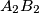$A_2B_2$ meet at$C$. Prove that, as$A_1$ and$B_1$ vary, the circumcentres of triangles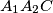$A_1A_2C$ all lie on one fixed circle.
Izvor: Međunarodna matematička olimpijada, shortlist 2002